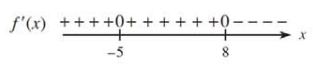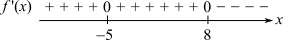Chapter 10.1, Problem 6E### Mathematical Applications for the ...

11th Edition
Ronald J. Harshbarger + 1 other
ISBN: 9781305108042

#### Solutions

Chapter
Section### Mathematical Applications for the ...

11th Edition
Ronald J. Harshbarger + 1 other
ISBN: 9781305108042
Textbook Problem

# In Problems 5 and 6, use the sign diagram for f ' ( x ) to determine (a) the critical values of f ( x ) , (b) intervals on which f ( x ) increases, (c) intervals on which f(x) decreases, (d) x − values at which relative maxima occur, and (c) x-values at which relative minima occur.(a)

To determine

The critical values of y=f(x), using the sign diagram of f(x):Explanation

Given Information:

The provided figure of the sign diagram of f(x):

Explanation:

Consider the given sign diagram:

The critical values are the only values at which the graph can have turning points, the derivative cannot change sign anywhere except at the critical value

(b)

To determine

The intervals on which y=f(x) increases, in the sign diagram as follows:(c)

To determine

The intervals on which y=f(x) decreases, in the sign diagram as follows:(d)

To determine

The x-values at which the relative maxima occurs, in the sign diagram as follows:(e)

To determine

The x-values at which the relative minima occurs, in the sign diagram as follows:### Still sussing out bartleby?

Check out a sample textbook solution.

See a sample solution

#### The Solution to Your Study Problems

Bartleby provides explanations to thousands of textbook problems written by our experts, many with advanced degrees!

Get Started

#### In Exercise 11-14, factor the expression. y23x+94

Calculus: An Applied Approach (MindTap Course List)

#### 0 1 ∞

Study Guide for Stewart's Multivariable Calculus, 8th

#### True or False: f(x) = tan x is differentiable at x=2.

Study Guide for Stewart's Single Variable Calculus: Early Transcendentals, 8th